# NCERT Solutions for Class 12 Chemistry Chapter 2 Solutions

Here we provide NCERT Solutions for Class 12 Chemistry Chapter 2 Solutions for English medium students, Which will very helpful for every student in their exams. Students can download the latest NCERT Solutions for Class 12 Chemistry Chapter 2 Solutions pdf, free NCERT solutions for Class 12 Chemistry Chapter 2 Solutions book pdf download. Now you will get step by step solution to each question.

## NCERT Solutions for Class 12 Chemistry Chapter 2 Solutions

Class 12 Chemistry Chapter 2 Solutions are defined as homogeneous mixture of two or more components. This chapter gives an overview of different types of solutions. Various laws and its derivations are provided here stepwise for easy understanding. The NCERT Solutions for Class 12 Chapter 2 provide solutions to the questions provided in the textbook.

NCERT IN-TEXT QUESTIONS

Question 1.
Calculate the mass percent of benzene (C6H6) and carbon tetrachloride (CCl4) if 22 g of benzene is dissolved in 122 g of carbon tetrachloride.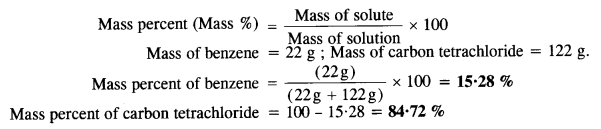Question 2.
Calculate the mole fraction of benzene in a solution containing 30% by mass of it in carbon tetrachloride.
Mass of benzene = 30 g
Mass of carbon tetrachloride = 70 g
Molar mass cf benzene (C6H6) = 6 x 12 + 6 x 1 = 78g mol-1.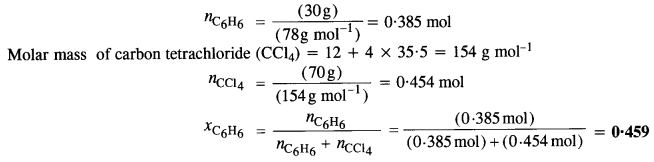Question 3.
Calculate the molarity of each of the following solutions:
(a) 30 g of CO(NO3)2.6H2O in 4.3 L of solution
(b) 30 mL of 0.5 M H2SO4 diluted to 500 mL.
(a) Molar mass of CO(NO3)2.6H2O=310.7 g mol-1
no. of moles = 30/310.7 = 0.0966
Vol. of solution = 4.3 L
Molarity =0.0966/4.3 = 0.022M
(b) 1000 mL of 0.5M  H2SO4 contain H2SO4 = 0.5 mole
30 mL of 0.5 M H2SO4 contain H2SO4
=0.5/1000 x 30 = 0.015 mole
Volume of solution = 500mL=0.5 L
Molarity = 0.015/0.5 = 0.03M

Question 4.
Calculate the mass of urea (NH2CONH2) required to prepare 2-5 kg of 0-25 molal aqueous solution.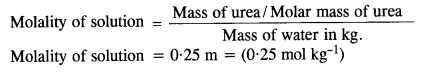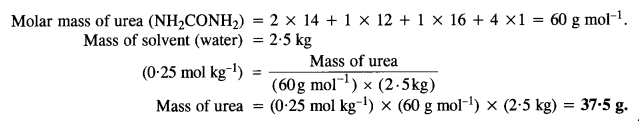Question 5.
Calculate (a) molality (b) molarity and (c) mole fraction of KI if the density of 20% (mass/mass) aqueous KI is 1.202 g mL-1.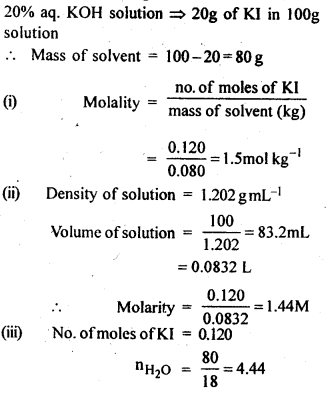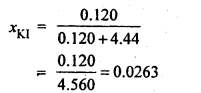Question 6.
H2S a toxic gas with rotten egg smell, is used for the qualitative analysis. If the solubility of H2S in water at S.T.P is 0·195 m ; calculate Henry’s law constant.
Step I. Calculation of mole fraction of H2S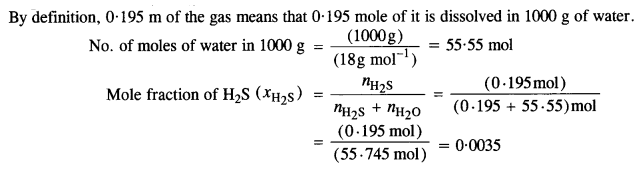Step II. Calculation of Henry’s Law constant
According to Henry’s Law,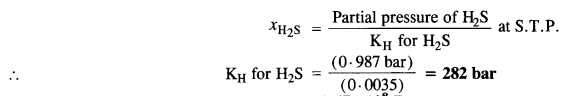Question 7.
Henry’s Law constant for CO2 in water is 1·67 x 108 Pa at 298 K. Calculate the quantity of CO2 in 500 mL of soda water when packed under 2·5 atm pressure of CO2 at 298 K. (D.S.B. 2008 Supp.)
Step I. Calculation of number of moles of CO2.
According to Henry’s Law,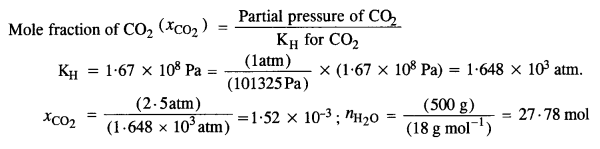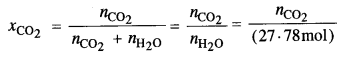(({ n }_{ { CO }_{ 2 } }) has been neglected as the gas is very little soluble in water)
∴ ({ n }_{ { CO }_{ 2 } }) = ({ x }_{ { CO }_{ 2 } }) x (27·78 mol) = (1·52 x 10-3) x (27·78 mol) = 0·0422 mol
Step II. Mass of CO2 dissolved in water = (0·0422 mol) x (44 g mol-1) = 1·857 g.

Question 8.
The vapour pressure of pure liquids A and B are 450 and 700 mm Hg respectively, at 350 K. Find out the composition of the liquid mixture if total vapour pressure is 600 mm Hg. Also find the composition of the vapour phase.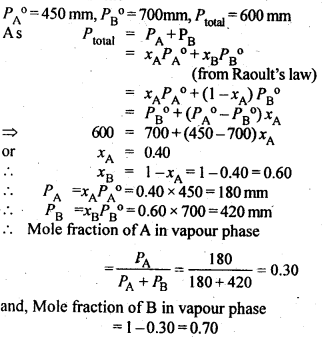Question 9.
The vapour pressure of pure water at 298 K is 23·8 mm Hg. 50 g of Urea (NH2CONH2) is added to 850 g of water. Calculate the vapour pressure of water for this solution and also its relative lowering in vapour pressure.
Step I. Calculation of vapour pressure of water for the solution
According to Raoult’s Law,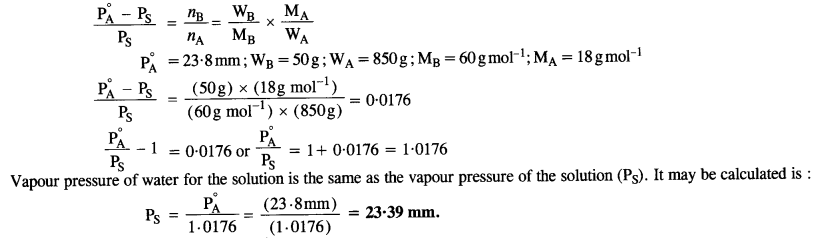Step II. Calculation of relative lowering in vapour pressure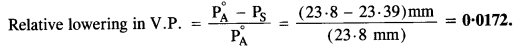Question 10.
Boiling point of water at 750 mm Hg is 99·63°C. How much sucrose is to be added to 500 g of water so that it may boil at 100°C ? (K6 for water = 0·52 K kg mol-1).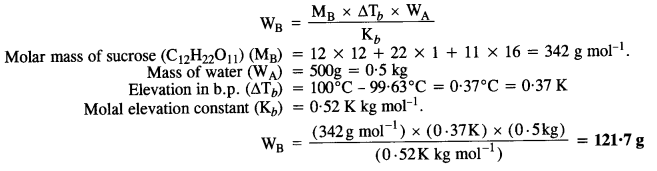Question 11.
Calculate the mass of ascorbic acid (Vitamin C, C6H8O6) to be dissolved in 75 g of acetic acid to lower its melting point by 1.5°C. Kf= 3.9 K kg mol-1.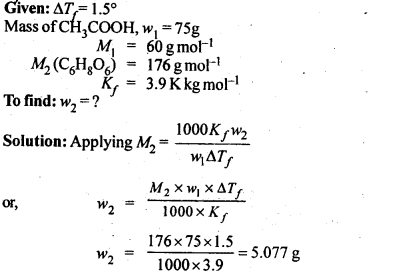Question 12.
Calculate the osmotic pressure in pascals exerted by a solution prepared by dissolving 1·0 g of a polymer of molar mass 185,000 in 450 mL of solution at 37°C.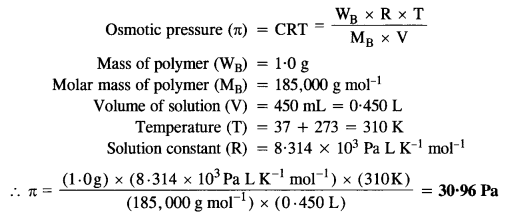NCERT EXERCISE

Question 1.
Define the term solution. What kinds of solutions are possible ? Write briefly about each type of solution with an example.
A solution may contain two or more substances also called components or constituents. A solution which has two components is known as binary solution (e.g. a solution of NaCl in water) while a solution with three components is called ternary solution (e.g. a solution of NaCl and KCl in water). Similarly even more than three components may also be present.
TYPES OF SOLUTION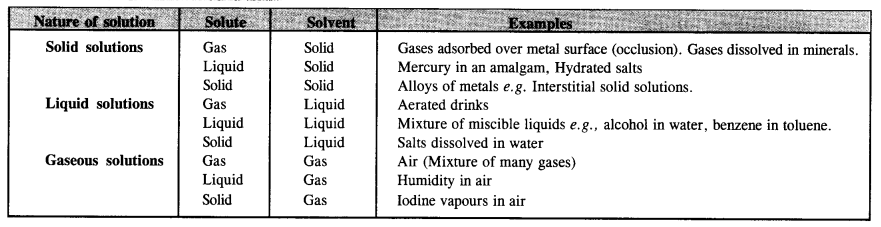Question 2.
Give an example of a solid solution in which the solute is a gas.
Solution of hydrogen in palladium and dissolved gases in minerals.

Question 3.
Define the following terms :
(i) Mole fraction
(ii) Molality
(iii) Molarity
(iv) Mass percentage.
(i) Mole fraction :
The ratio of the number of moles of one component to the total number of moles of all the components present in the solution.
For a binary solution made up of components A and B,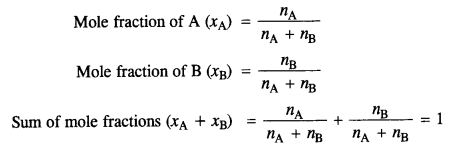(ii) Molality:
The number of gram moles of the solute dissolved in 1000 g (or kg) of the solvent.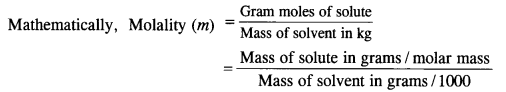(iii) Molarity:
The number of gram formula mass of the solute dissolved per litre of the solution.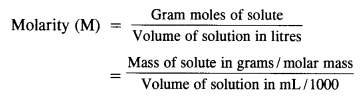(iv) Mass percentage:
The number of parts by mass of one component (solute or solvent) per 100 parts by mass of the solution. If A and B are the two components of a binary solution,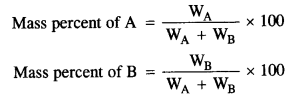Question 4.
Concentrated nitric acid used in laboratory work is 68% nitric acid by mass in aqueous solution. What should be the molarity of such a sample of the acid if the density of the solution is 1.504 g mL-1?
68% nitric acid by mass means that 68g mass of nitric acid is dissolved in 100g mass of solution. Molar mass of HNO3= 63g mol-1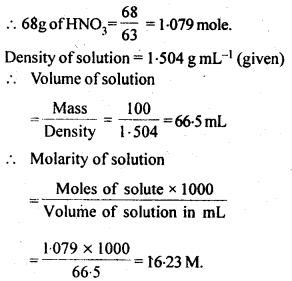Question 5.
A solution of glucose in water is labelled as 10 percent W/W. What would be the molality and mole fraction of each component in the solution ? If the density of the solution is 1·2 g mL-1, then what should be the molarity of the solution ? (C.B.S.E. 2013, Manipur Board 2015)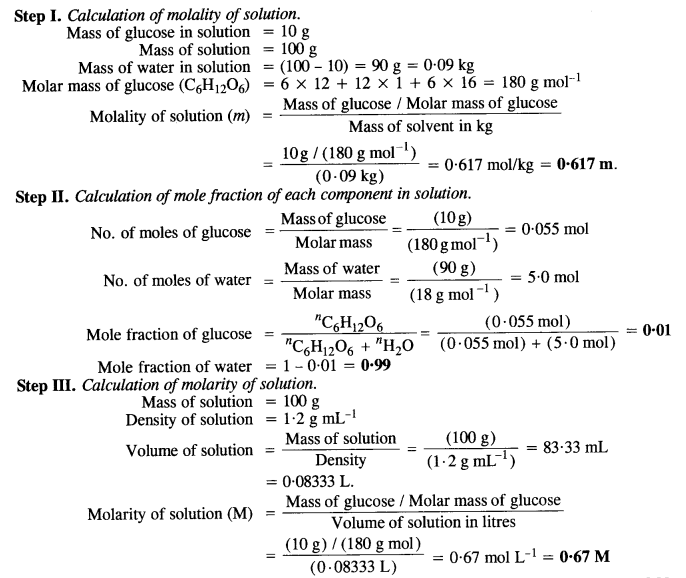Question 6.
How many mL of a 0·1 M HCl are required to react completely with lg mixture of Na2CO3 and NaHCO3 containing equimolar amounts of two ?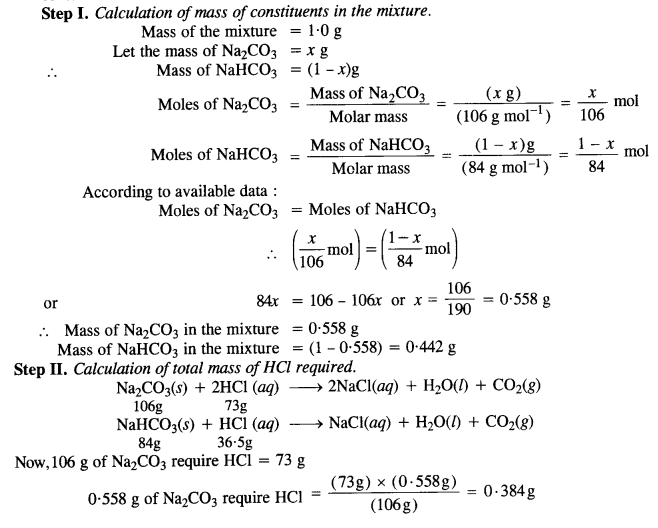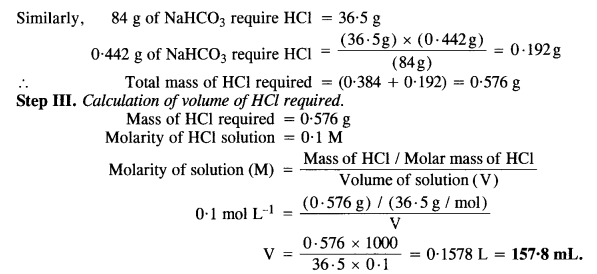Question 7.
A solution is obtained by mixing 300 g of 25% solution and 400 g of 40% solution by mass. Calculate the mass percentage of the resulting solution.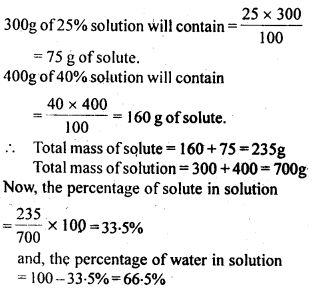Question 8.
An antifreeze solution is prepared from 222·6 g of ethylene glycol C2H4(OH)2 and 200 g of water. Calculate the molality of the solution. If the density of the solution is 1·072 g mL-1, then what shall be the molarity of the solution? (C.B.S.E. Delhi 2007)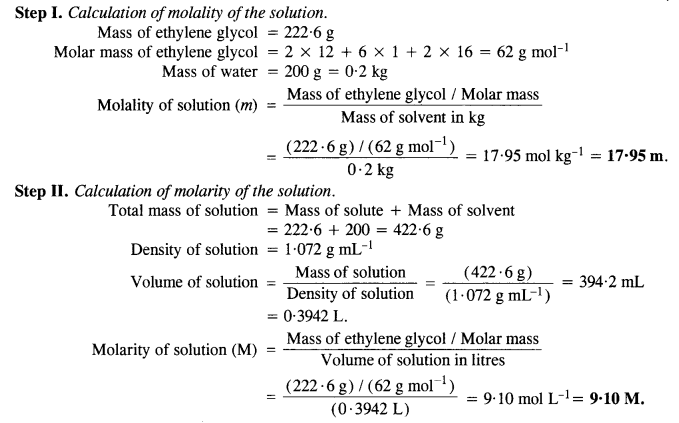Question 9.
A sample of drinking water was found to be severely contaminated with chloroform(CHCl3) supposed to be carcinogen. The level of contamination was 15 ppm (by mass).
(i) Express this in percent by mass.
(ii) Determine the molality of chloroform in water sample.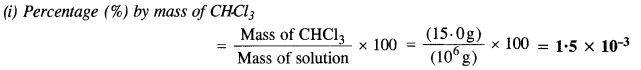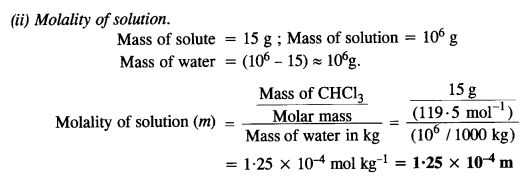Question 10.
What role does the molecular interaction play in a solution of alcohol and water?
Alcohol and water both have strong tendency to form intermolecular hydrogen bonding. On mixing the two, a solution is formed as a result of formation of H-bonds between alcohol and H2 O molecules but these interactions are weaker and less extensive than those in pure H2O. Thus they show a positive deviation from ideal behaviour. As a result of this, the solution of alcohol and water will have higher vapour pressure and lower boiling point than that of water and alcohol.

Question 11.
Why do gases nearly always tend to be less soluble in liquids as the temperature is raised ?
The dissolution of a gas in a liquid is exothermic in nature because the gas contracts in volume.

Gas + Liquid ⇌ Dissolved gas ; ∆H = – ve

An increase in temperature will favour the reverse process since it is of endothermic nature. Therefore, the solubility of the gas in solution decreases with the rise in temperature.

Question 12.
State Henry’s law and mention some important applications.
The effect of pressure on the solubility of a gas in a liquid is governed by Henry’s Law. It states that the solubility of a gas in a liquid at a given temperature is directly proportional to the partial pressure of the gas Mathematically, P = KHX where P is the partial pressure of the gas; and X is the mole fraction of the gas in the solution and KH is Henry’s Law constant.
Applications of Henry’s law
(i) In the production of carbonated beverages (as solubility of C02 increases at high pressure).
(ii) In the deep sea diving.
(iii) For climbers or people living at high altitudes, where low blood 02 causes climbers to become weak and make them unable to think clearly

Question 12.
State Henry’s law and mention some of its important applications.
Henry’s law: The solubility of a gas in a liquid at a particular temperature is directly proportional to the pressure of the gas in equilibrium with the liquid at that temperature.
or
The partial pressure of a gas in vapour phase is proportional to the mole fraction of the gas (x) in the solution. p = KHX
where KH is Henry’s law constant.
Applications of Henry’s law :
(i) In order to increase the solubility of CO2 gas in soft drinks and soda water, the bottles are normally sealed under high pressure. Increase in pressure increases the solubility of a gas in a solvent according to Henry’s Law. If the bottle is opened by removing the stopper or seal, the pressure on the surface of the gas will suddenly decrease. This will cause a decrease in the solubility of the gas in the liquid i.e. water. As a result, it will rush out of the bottle producing a hissing noise or with a fiz.
(ii) As pointed above, oxygen to be used by deep sea divers is generally diluted with helium inorder to reduce or minimise the painfril effects during decompression.
(iii) As the partial pressure of oxygen in air is high, in lungs it combines with haemoglobin to form oxyhaemoglobin. In tissues, the partial pressure of oxygen is comparatively low. Therefore, oxyhaemoglobin releases oxygen in order to carry out cellular activities.

Question 13.
The partial pressure over a saturated solution containing 6·56 x 10-2 g of ethane is 1 bar. If the solution contains 5·0 x 10-2 g of ethane, what shall be the partial pressure of the gas ?
According to Henry’s law,
The mass of the gas (m) dissolved in solution ∝ Partial pressure (p) (At constant temperature)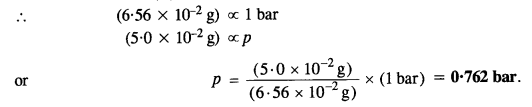Question 14.
What is meant by positive and negative deviations from Raoult’s law and how is the sign of ∆Hsol related to positive and negative deviations from Raoult’s law ?
(a) Positive Deviation:
(i) ∆Vmixing is positive: This is quite likely also because in ge presence of weak forces of interaction, the interaction, the volume of the solution is bound to increase.
(ii) ∆Hmixing is positive: Energy is needed to form the solution because the components of the solution have to be brought closer to form the solution. Thus, the process of mixing is of endothermic nature.

(b) Negative Deviation:

(i) ∆Vmixing is negative: Because of the increased forces of interaction, the molecules of the two components will come closer and as a result, there is a decrease in the volume of the solution.
(ii) ∆Hmixing is negative: Energy is expected to be released because of the increase in the forces of interaction. Therefore, the process of mixing is exothermic in nature or ∆Hmixing is negative.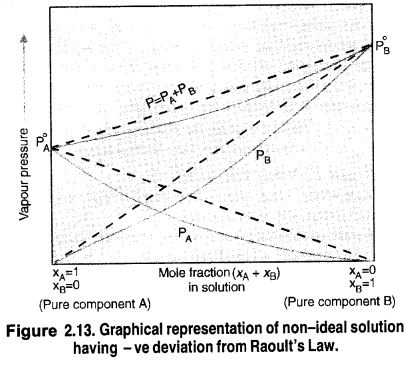Question 15.
An aqueous solution of 2% non-volatile solute exerts a pressure of 1.004 bar at the normal boiling point of the solvent. What is the molar mass of the solute?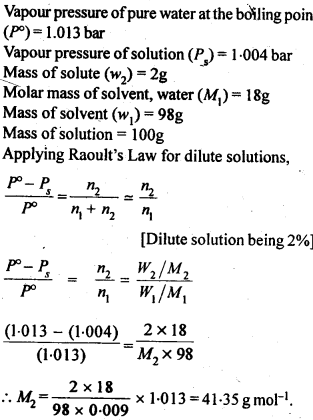Question 16.
Heptane and octane form ideal solution. At 373 K, the vapour pressure of the two liquid components are 105·2 k Pa and 46·8 k Pa respectively. If the solution contains 25 g of heptane and 35 g of octane, calculate :
(i) Vapour pressure exerted by heptane
(ii) Vapour pressure exerted by octane
(iii) Vapour pressure exerted by the solution
(iv) Mole fraction of octane in the vapour phase. (C.B.S.E. Sample Paper, 2010)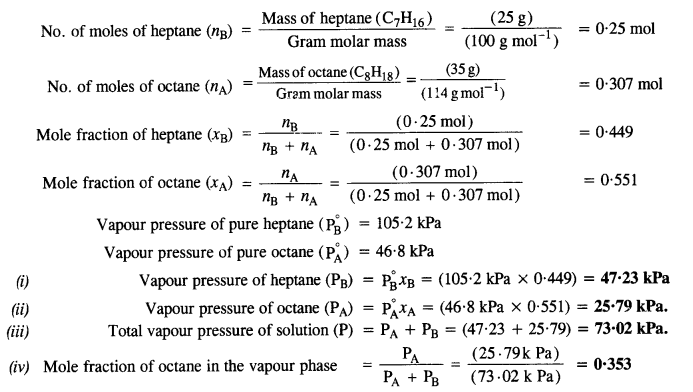Question 17.
The vapour pressure of water is 12·3 kPa at 300 K. Calculate the vapour pressure of 1 molal solution in it.
1 molal solution implies one mole of the solute dissolved in 1000 g (1 kg) of solvent i.e. water.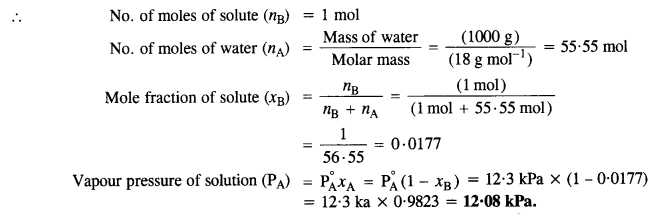Question 18.
Calculate the mass of a non-volatile solute (molar mass 40 g mol-1) which should be dissolved in 114 g octane to reduce its vapour pressure to 80%.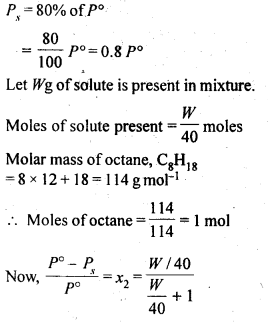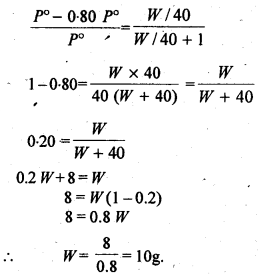Question 19.
A solution containing 30 g of a non-volatile solute exactly in 90 g of water has a vapour pressure of 2·8 k Pa at 298 K. Further 18 g of water is then added to the solution and the new vapour pressure becomes 2·9 k Pa at 298 K. Calculate
(i) Molecular mass of the solute.
(ii) Vapour pressure of water at 298 K. (C.B.S.E. Outside Delhi 2005)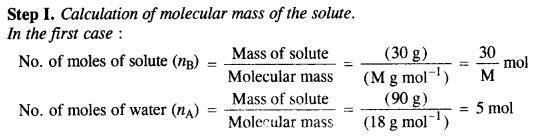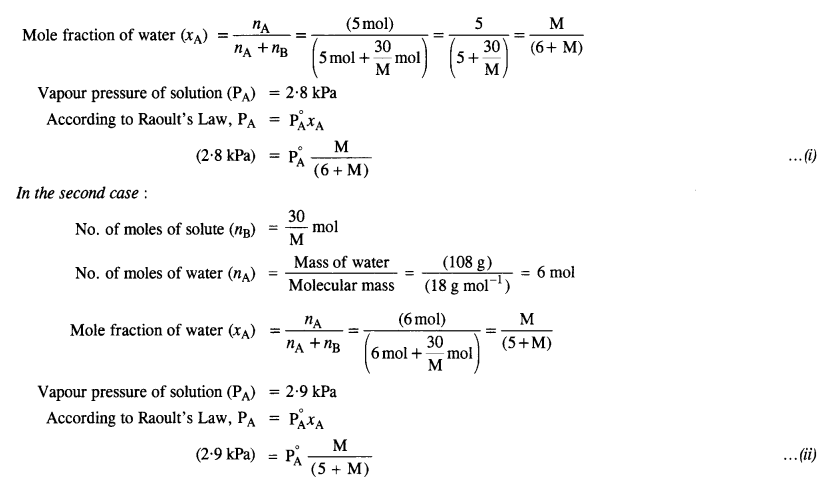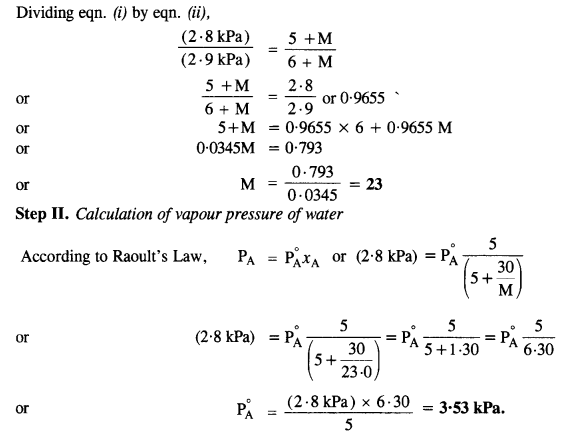Question 20.
A 5% solution (by mass) of cane sugar in water has freezing point of 271 K. Calculate the freezing point of a 5% glucose in water if the freezing point of pure water is 273·15 K. (C.B.S.E. Delhi 2008)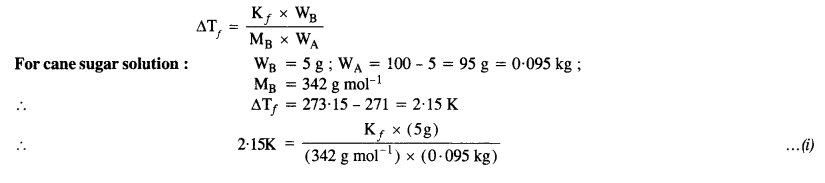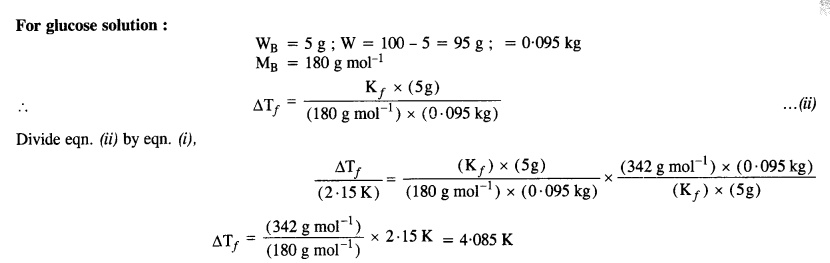Freezing point temperature of glucose solution = (273·15 – 4·085) K = 269·07 K.

Question 21.
Two elements A and B form compounds having molecular formulae AB2 and AB4. When dissolved in 20 g of benzene, 1 g of AB2 lowers the freezing point by 2·3 K whereas 1 g of AB4 lowers it by 1·3 K. Molal depression constant for benzene is 5·1 K kg mol-1. Calculate atomic masses of A and B. (C.B.S.E. Delhi 2004)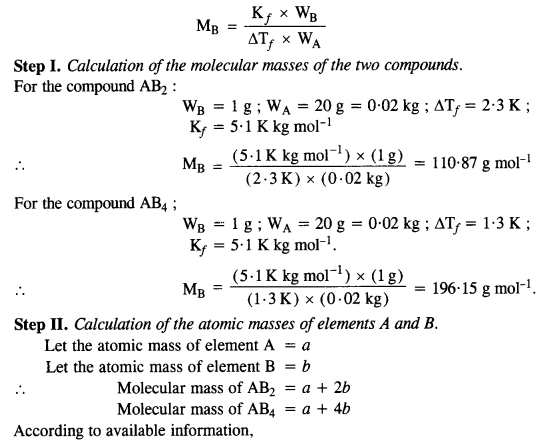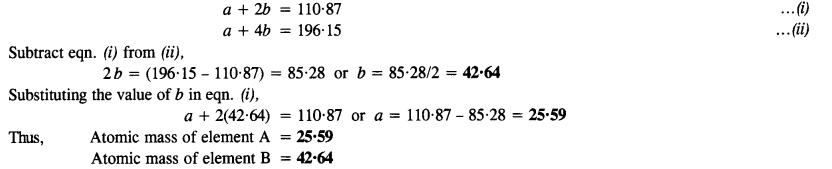Question 22.
At 300 K, 36g of glucose present in a litre of its solution has an osmotic pressure of 4.08 bar. If the osmotic pressure of the solution is 1.52 bars at the same temperature, what would be its concentration?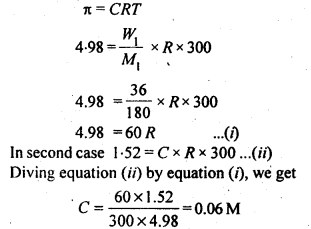Question 23.
Suggest the most important type of intermolecular attractive interactions in the following pairs :
(i) n-hexane and n-octane
(ii) I2 and CCl4
(iii) NaClO4 and water (H2O)
(iv) methanol and acetone
(v) acetonitrile (CH3CN) and acetone (C3H6O).
(i) London’s forces
(ii) London’s forces
(iii) Ion-dipole interactions
(iv) Intermolecular hydrogen bonding
(v) Dipole- dipole interactions.

Question 24.
Based on solute-solvent interactions, arrange the following in order of increasing solubility in n-octanc and explain.
Cyclohexane, KCl, CH3OH, CH3CN.
(a) Cyclohexane and n-octane both are non-polar. They mix completely in all proportions.
(b) KCl is an ionic compound, KCl will not dissolve in n-octane.
(c) CH3OH is polar. CH3OH will dissolve in n-octane.
(d) CH3CN is polar but lesser than CH3OH. Therefore, it will dissolve in n-octane but to a greater extent as compared to CH3OH. Hence, the order is KCl < CH3OH < CH3CN < Cyclohexane.

Question 25.
Among the following compounds, identify which are insoluble, partially soluble and highly soluble in water ?
(i) phenol
(ii) toluene
(iii) formic acid
(iv) ethylene glycol
(v) chloroform
(vi) pentanol.
(i) phenol (C6H5OH): Is partially soluble in water due to weak dipole-dipole interactions in the molecules of phenol and water.
(ii) toluene (C7H8) : Is insoluble in water because it is an aromatic hydrocarbon (non-polar) while water is polar in nature.
(iii) formic acid (HCOOH) : Is highly soluble in water since it can form hydrogen bending with water.
(iv) ethylene glycol (HOCH2CH2OH) : Is highly soluble in water since it can form hydrogen bonding with water.
(v) chloroform (CHCl3): Is insoluble in water because it is an organic heavy liquid and forms a separate layer.
(vi) pentanol (C5H11OH) : In partially soluble in water because the bulky C5H11 group decreases its extent of hydrogen bonding with water.

Question 26.
If the density of some lake water is 1.25 g mL-1 and contains 92g of Na+ ions per kg of water, calculate the molality of Na+ ions in the lake.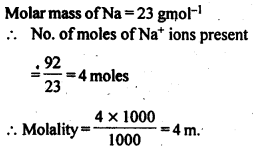Question 27.
If the solubility product of CuS is 6 x 10-16, calculate the maximum molarity of CuS in aqueous solution.
Dissociation of CuS in aqueous solution is :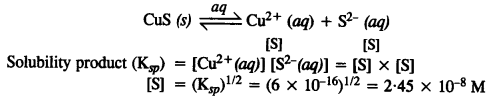By definition Ksp corresponds to the product of ionic concentration of the salt in saturated solution and it represents maximum molarity of the salt. Therefore, maximum molarity of the salt = 2· 45 x 10-8 M.

Question 28.
Calculate the mass percent of aspirin (C9H8O4) in acetonitrile (CH3CN) when 6·5 g of aspirin is dissolved in 450 g of CH3CN.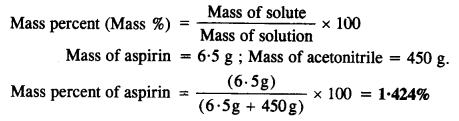Question 29.
Nalorphene (C19H21NO3) similar to morphine is used to combat withdrawal symptoms in narcotic users. Dose of nalorphene generally given is 1· 5 mg. Calculate the mass of 1· 5 x 10-3 m aqueous solution required for the above doze ?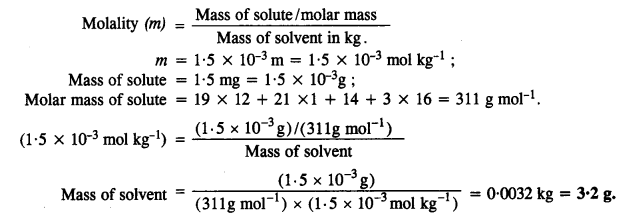Question 30.
Calculate the amount of benzoic acid (C6H5COOH) required for preparing 250 mL of 0.15 M solution in methanol 0.15 M solution means than 0.15 mole of benzoic acid is dissolved in 1L of solution.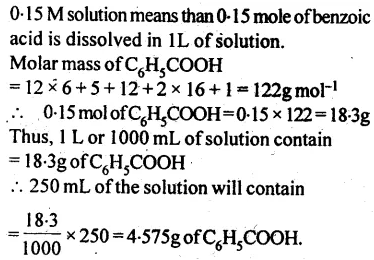Question 31.
The depression in freezing point of water observed for the same amount of acetic acid, trichloroactetic acid and trifluoroacetic acid increases in the order given above. Explain. (C.B.S.E. 2008 Supp.)
The depression in freezing point of a solute in water depends upon the number of particles or ions furnished by it in solution or upon its degree of dissociation (α). All the three organic acids ionise in aqueous solution. However, the relative order of acidic strengths is as given below.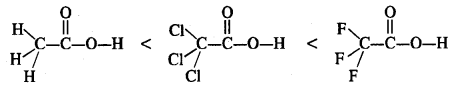This is linked with the electronegativity of the halogen atoms present. Fluorine (F) is more electronegative than (Cl). Under the circumstances, trifluoroacetic acid gives maximum ions in solution since it is the strongest acid. Consequently, the depression in freezing point (∆Tf) is the maximum in this case and is the least for acetic acid which is the weakest acid.

Question 32.
Calculate the depression in freezing point of water when 10 g of CH3CH2CH(Cl)COOH is added to 250 g of water. Ka = 1·4 x 10-3; Kf = 1·86 K kg mol-1. (C.B.S.E. 2008 Supp.)
Step I. Calculation of degree of dissociation of acid Mass of acid = 10 g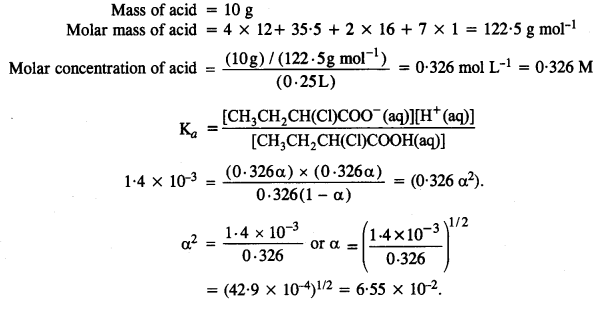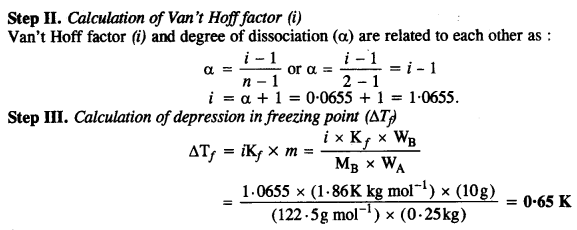Question 33.
19·5 g of CH3FCOOH is dissolved in 500 g of water. The depression in the freezing point of water observed is 1·0°C. Calculate Van’t Hoff factor and dissociation constant of the acid : Kf = 1·86 K kg mol-1.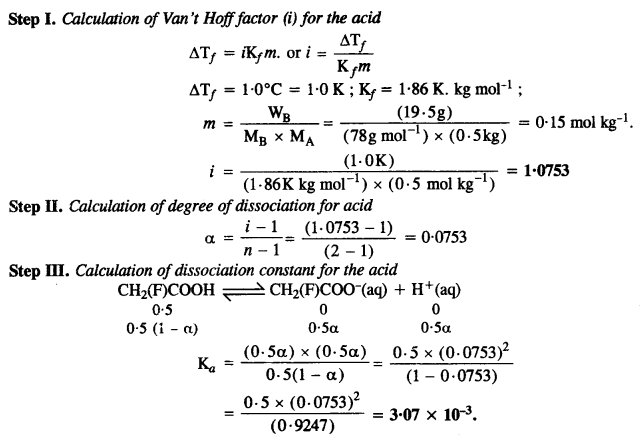Question 36.
100 g of liquid A (molar mass 140 g mol-1) was dissolved in 1000 g of liquid B (molar mass 180 g mol-1). The vapour pressure of pure liquid B was found to be 500 torr. Calculate the vapour pressure of pure liquid A and its vapour pressure in the solution if the total vapour pressure of the solution is 475 torr.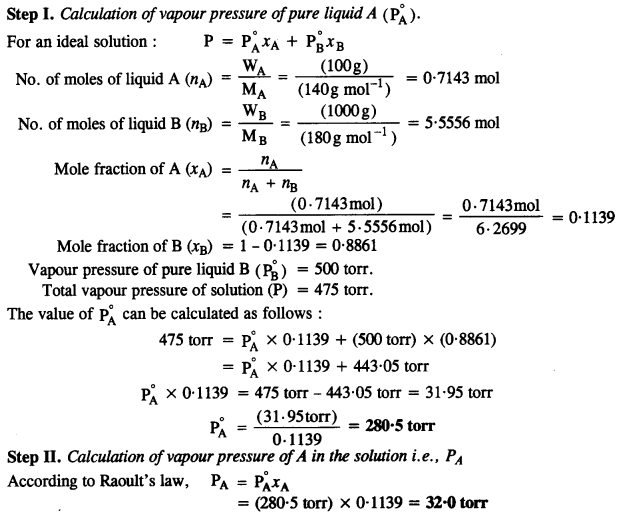Question 37.
Vapour pressures of pure acetone and chloroform at 328 K are 632·8 mm Hg and 741·8 mm Hg respectively. Assuming that they form ideal solution over die entire range of composition, plot ptotal. pchloroform, and pacetone as a function of xacetone. The experimental data observed for different compositions of mixture is :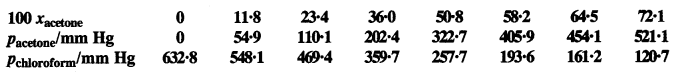Plot this data also on the same graph paper. Indicate whether it has positive deviation or negative the ideal solution.
From the available information :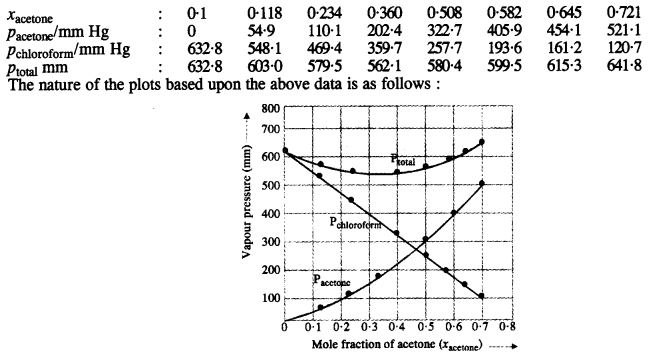Since the plot or graph dips downwards, the solution shows negative deviation from Raoult’s Law.

Question 38.
Benzene and naphthalene form ideal solution over the entire range of composition. The vapour pressure of pure benzene and naphthalene at 300 K are 50·71 mm Hg and 32·06 mm Hg respectively. Calculate the mole pure fraction of benzene in vapour phase if 80 g of benzene is mixed with 100 g of naphthalene.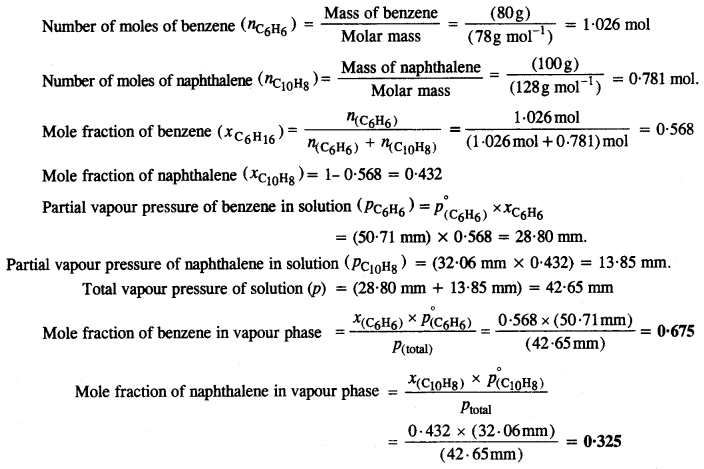Question 39.
Air is a mixture of number of gases. The major components are oxygen and nitrogen with approximate proportion of 20% is to 79% by volume at 298 K. The water is in equilibrium with air at a pressure of 10 atm. At 298 K if the Henry’s law constants for oxygen and nitrogen at 298 K are 3·30 x 107 mm and 6·51 x 107 mm respectively, calculate the composition of these gases in water.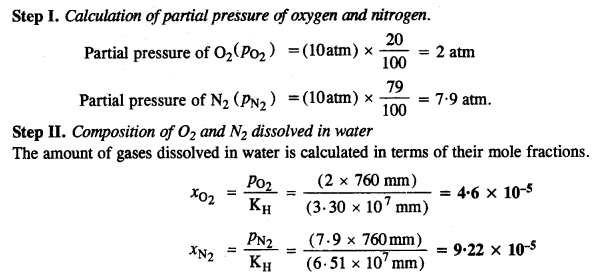Question 40.
Determine the amount of CaCl2 (i = 2·47) dissolved in 2·5 litre of water so that its osmotic pressure is 0·75 atm at 27°C.
According to Van’t Hoff equation :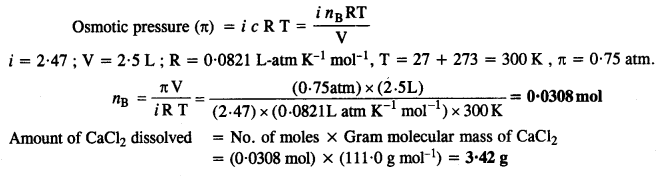Question 41.
Determine the osmotic pressure of a solution prepared by dissolving 25 mg of K2SO4 in 2 litre of water at 25°C, asstiming that it is completely dissociated.Answer: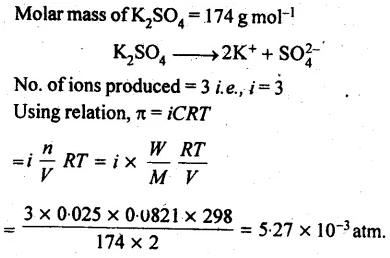All Chapter NCERT Solutions For Class 12 Chemistry

—————————————————————————–

All Subject NCERT Solutions For Class 12

*************************************************

I think you got complete solutions for this chapter. If You have any queries regarding this chapter, please comment on the below section our subject teacher will answer you. We tried our best to give complete solutions so you got good marks in your exam.

If these solutions have helped you, you can also share ncertsolutionsfor.com to your friends.